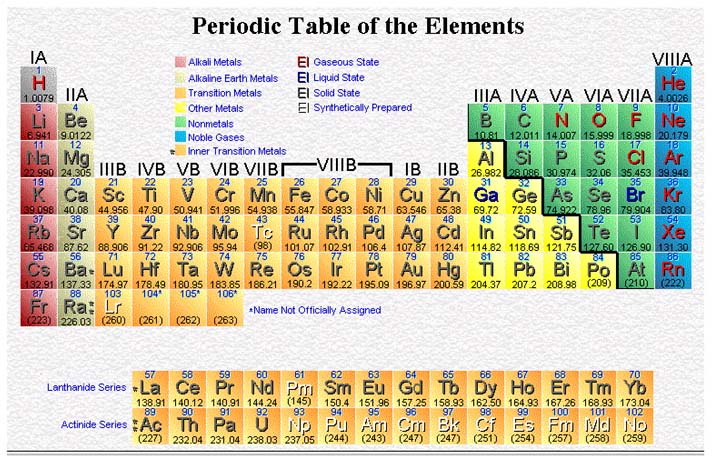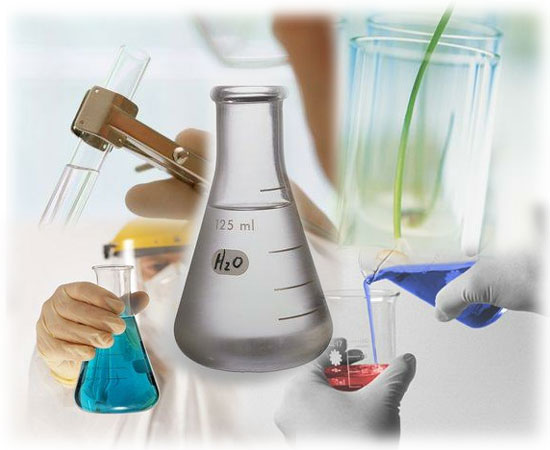CHEMISTRY Pre-AP Unit 7: Chemical Reactions Chapter 10Goal: To understand how to write and balance chemical equations and to predict the products when given the reactants.

Objectives: The student should be able to:

1. Identify the reactants and products in a chemical equation.

2. From the word equation for a chemical reaction, write and balance the formula equation for the reaction.

3. Use appropriate physical state symbols for the reactants and products when the physical state is known.

4. Identify a chemical reaction as one of the five major reaction types.

5. When given a set of reactants and reaction conditions, predict whether or not a chemical reaction will occur. If a reaction does occur, complete an equation (word or formula) showing the product(s) formed.

Activities:

Nov 7: Discuss Unit V Exam; Begin Lecture: Balancing Equations

Nov 8: Finish Lecture: Balancing Equations

Nov 9: Quiz: Balancing Equations; Lecture: Reaction Types

Nov 10: Discuss Reaction Types Homework; Finish Lecture: Reaction Types

Nov 13: Quiz: Reaction Types; Discuss Predicting Products Homework

Nov 14: Lab: Reaction Types

Nov 15: Teacher Demonstrations

Nov 16: Quiz: Predicting Products; Review

Nov 17: Unit VII Exam

Homework:

Nov 7: Balancing Equations HW

Nov 8: Study for Balancing Equations Quiz

Nov 9: Reaction Types HW

Nov 10: Study for Reaction Types Quiz; Begin Predicting Products HW

Nov 13: Prelab

Nov 15: Study for Predicting Products Quiz

Nov 16: Study for Exam

Lecture & Lab Materials (uploaded once per week):

Balancing Equations

Reaction Types